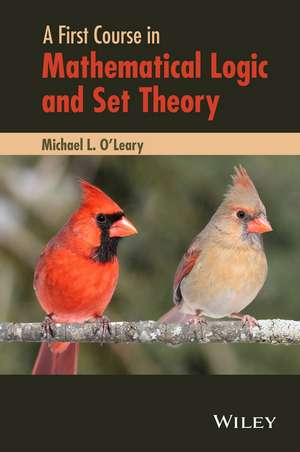# A First Course in Mathematical Logic and Set Theory

## matematica si stiinte matematica fundatiile matematicii logica matematica

5 Review(s)
A mathematical introduction to the theory and applications of logic and set theory with an emphasis on writing proofs Highlighting the applications and notations of basic mathematical concepts within the framework of logic and set theory, A First Cou...
Cod: 9780470905883 / 314304
Disponibilitate: In stoc
Producator:

## 424.86 RON

A mathematical introduction to the theory and applications of logic and set theory with an emphasis on writing proofs Highlighting the applications and notations of basic mathematical concepts within the framework of logic and set theory, A First Course in Mathematical Logic and Set Theory...
Opinia ta despre produs

Produse din Categorie